#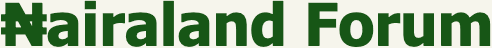Welcome, Guest: Join Nairaland / LOGIN! / Trending / Recent / New
Stats: 2,756,124 members, 6,548,588 topics. Date: Thursday, 21 October 2021 at 07:26 AM

## I.Q TEST: Let's See How Sound You Are In Quantitative Reasoning - Family (3) - Nairaland

(1) (2) (3) (4) (5) (Reply) (Go Down)

 Re: I.Q TEST: Let's See How Sound You Are In Quantitative Reasoning by PrinxArthur1(m): 6:48pm On Apr 12, 2020 Epositive:There's some "tiny blue stuffs" on the car. He is right I have seen it Re: I.Q TEST: Let's See How Sound You Are In Quantitative Reasoning by ADUKKY(f): 6:49pm On Apr 12, 2020 We dey wait your answer o.matrix199:I'll reveal the answers to pix 1 and pix 2 and acknowledge those who got it right later on. cc: RoyalRoyModified: I'm a very discreet person. The term 'acknowledge' means more than you might think. You never can tell! Re: I.Q TEST: Let's See How Sound You Are In Quantitative Reasoning by TheVerdict: 6:50pm On Apr 12, 2020 1. 402. 75 1 Like Re: I.Q TEST: Let's See How Sound You Are In Quantitative Reasoning by Blackbelly(m): 6:53pm On Apr 12, 2020 Epositive: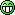I keep refreshing this page. Local man is anxious.Re: I.Q TEST: Let's See How Sound You Are In Quantitative Reasoning by Blackbelly(m): 6:55pm On Apr 12, 2020 These puzzles have more to do with observation prowess than numerical calculations.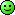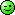Re: I.Q TEST: Let's See How Sound You Are In Quantitative Reasoning by PrinxArthur1(m): 6:58pm On Apr 12, 2020 Blackbelly:These puzzles have more to do with observation prowess than numerical calculations. You'll see in time.I have seen it, but the one that baffled me was the racing car with a tiny helmet, man l! Re: I.Q TEST: Let's See How Sound You Are In Quantitative Reasoning by Hallenjay: 7:38pm On Apr 12, 2020 Blackbelly:OP hope you won't leave us hanging? Some of us are dying of curiosity. op too is waiting for the real owner of d post to answer and provide proof before e can come here. He's online but cannot talk for now, am sure I can't go wrong wt my ansa because my prove is beyond every reasonable doubt Re: I.Q TEST: Let's See How Sound You Are In Quantitative Reasoning by Epositive(m): 7:44pm On Apr 12, 2020 Hallenjay: op too is waiting for the real owner of d post to answer and provide proof before e can come here. He's online but cannot talk for now, am sure I can't go wrong wt my ansa because my prove is beyond every reasonable doubt Osheeeeey Pascal!1 Like Re: I.Q TEST: Let's See How Sound You Are In Quantitative Reasoning by martjay(m): 7:54pm On Apr 12, 2020 First puzzle is 30Second puzzle is 152 Re: I.Q TEST: Let's See How Sound You Are In Quantitative Reasoning by matrix199(m): 8:17pm On Apr 12, 2020 Yea I'm back. Sorry for the delay. I had to give extra-time because no-one got both answers correctly. Only nine persons got one of the puzzles Re: I.Q TEST: Let's See How Sound You Are In Quantitative Reasoning by Flamez01(m): 8:21pm On Apr 12, 2020 1----202----43 Re: I.Q TEST: Let's See How Sound You Are In Quantitative Reasoning by StrikeBack(m): 8:22pm On Apr 12, 2020 1 50 2. 120 Re: I.Q TEST: Let's See How Sound You Are In Quantitative Reasoning by matrix199(m): 8:23pm On Apr 12, 2020 let's keep it coming Re: I.Q TEST: Let's See How Sound You Are In Quantitative Reasoning by StrikeBack(m): 8:24pm On Apr 12, 2020 1. 1002. 232 Re: I.Q TEST: Let's See How Sound You Are In Quantitative Reasoning by TheVerdict: 8:30pm On Apr 12, 2020 matrix199:I.Q challenge. Let's see how sound you are in quantitative reasoning. Here are two I.Q Trivia Puzzles...... 1. 402. 73Final Answer Re: I.Q TEST: Let's See How Sound You Are In Quantitative Reasoning by matrix199(m): 8:34pm On Apr 12, 2020 Well, I was hoping the trivia would hit the front page, so many folks would try out the puzzles. I was about saying the mods here are fond of posting irrelevant threads to front page, but it occurred to me to give them the benefits of doubt. Maybe they didn't get to see/know about the thead.As it stands, only two persons got the answer to one of the I.Q puzzles. Let me disclose the answers to the puzzles. Re: I.Q TEST: Let's See How Sound You Are In Quantitative Reasoning by matrix199(m): 8:39pm On Apr 12, 2020 check the front page. The second post. I'll modify and post the answers there Re: I.Q TEST: Let's See How Sound You Are In Quantitative Reasoning by babyfaceafrica: 9:04pm On Apr 12, 2020 matrix199:Well, I was hoping the trivia would hit the front page, so many folks would try out the puzzles. I was about saying the mods here are fond of posting irrelevant threads to front page, but it occurred to me to give them the benefits of doubt. Maybe they didn't get to see/know about the thead.As it stands, only two persons got the answer to one of the I.Q puzzles. Let me disclose the answers to the puzzles. Forget front page.. Who ẹ help? .. Also u didn't call the supermods.. How you want make your post make Frontpage? .. Also brainteasers don't generally make front page Re: I.Q TEST: Let's See How Sound You Are In Quantitative Reasoning by Aurelius1(m): 9:06pm On Apr 12, 2020 Trivia1: 30 Trivia2: 39 Re: I.Q TEST: Let's See How Sound You Are In Quantitative Reasoning by matrix199(m): 9:10pm On Apr 12, 2020 For picture 1; let x represents the helmet. It implies that x + x + x = 30 3x = 30 x = 30/3 = 10 so x is 10For picture 2, let y represents the car. It implies that y + y+ x = 20 since x is 10 y + y + 10 = 20 2y + 10 = 20 2y = 20 - 10 2y = 10 y = 10/2 = 5 so y is 5For picture 3, let z represents the pair of trophies( 2 trophies). It implies that z + z + y = 13 since y is 5 z + z + 5 = 13 2z + 5 = 13 2z = 13 - 5 2z = 8 z = 8/2 = 4 so z is 4 But remember that z is a pair of trophies It implies that 1 trophy is 4/2 = 2For Picture 4, x(10) + y(5) x 1/2 of z(2) _____ 10 + 5 x 2Apply BODMAS _______________ 10 + (5 x 2) 10 + 10 = 20.Puzzle 2Take a good look at each quantitative picture. The 1st picture clearly shows 7 petals in each flower pot(7 + 7 + 7 = 21), discreetly indicating that each petal is 1. The 2nd picture also shows 4 roses in each flower pot. it implies that ( 4x2 + 4x2 + 4x2 =8 + 8 + 8= 24 or 12x2 = 24). The 3rd picture indicates that each empty pot is 4, that is ( 4 + 4 + 4 = 12). Now, the 4th picture shows a flower pot of 6 petals( each petal is 1, totalling 6 in combined value) + an empty flower pot having a value of 4 x a flower pot of 5 roses having a combined value of 10(since each rose is 2 in value) That is, 6 + 4 x 10 Don't forget to apply "BODMAS" 6 + (4 x 10) 6 + 40 = 46. Thus, for puzzle 1, the answer is 20! for puzzle 2, the answer is 46! Re: I.Q TEST: Let's See How Sound You Are In Quantitative Reasoning by Taymi: 9:18pm On Apr 12, 2020 matrix199:For picture 1; let x represents the helmet. It implies that x + x + x = 30 3x = 30 x = 30/3 = 10 so x is 10For picture 2, let y represents the car. It implies that y + y+ x = 20 since x is 10 y + y + 10 = 20 2y + 10 = 20 2y = 20 - 10 2y = 10 y = 10/2 = 5 so y is 5For picture 3, let z represents the pair of trophies( 2 trophies). It implies that z + z + y = 13 since y is 5 z + z + 5 = 13 2z + 5 = 13 2z = 13 - 5 2z = 8 z = 8/2 = 4 so z is 4 But remember that z is a pair of trophies It implies that 1 trophy is 4/2 = 2For Picture 4, x(10) + y(5) x 1/2 of z(2) _____ 10 + 5 x 2Apply BODMAS _______________ 10 + (5 x 2) 10 + 10 = 20.So what happen to that blue thing in the car that makes it different from other cars 1 Like Re: I.Q TEST: Let's See How Sound You Are In Quantitative Reasoning by ADUKKY(f): 9:19pm On Apr 12, 2020 matrix199:For picture 1; let x represents the helmet. It implies that x + x + x = 30 3x = 30 x = 30/3 = 10 so x is 10For picture 2, let y represents the car. It implies that y + y+ x = 20 since x is 10 y + y + 10 = 20 2y + 10 = 20 2y = 20 - 10 2y = 10 y = 10/2 = 5 so y is 5For picture 3, let z represents the pair of trophies( 2 trophies). It implies that z + z + y = 13 since y is 5 z + z + 5 = 13 2z + 5 = 13 2z = 13 - 5 2z = 8 z = 8/2 = 4 so z is 4 But remember that z is a pair of trophies It implies that 1 trophy is 4/2 = 2For Picture 4, x(10) + y(5) x 1/2 of z(2) _____ 10 + 5 x 2Apply BODMAS _______________ 10 + (5 x 2) 10 + 10 = 20.You can't ignore the helmet in the car.....it wasn't in the other cars Re: I.Q TEST: Let's See How Sound You Are In Quantitative Reasoning by matrix199(m): 9:23pm On Apr 12, 2020 ADUKKY:You can't ignore the helmet in the car.....it wasn't in the other carsHave you heard of decoy? Well, that was a decoy, because no mathematical operation was affixed to the differed picture. Re: I.Q TEST: Let's See How Sound You Are In Quantitative Reasoning by ADUKKY(f): 9:27pm On Apr 12, 2020 matrix199:Have you heard of decoy? Well, that was a decoy, because no mathematical operation was affixed to the differed picture. I think this is more of logical reasoning question and not mathematics.....I suggest you check your answer again to ensure you give people the right perspective. Re: I.Q TEST: Let's See How Sound You Are In Quantitative Reasoning by Taymi: 9:30pm On Apr 12, 2020 ADUKKY: I think this is more of logical reasoning question and not mathematics.....I suggest you check your answer again to ensure you give people the right perspective.His solution is thrash Check the solution for the second puzzle on the first page. Re: I.Q TEST: Let's See How Sound You Are In Quantitative Reasoning by matrix199(m): 9:32pm On Apr 12, 2020 ADUKKY: I think this is more of logical reasoning question and not mathematics.....I suggest you check your answer again to ensure you give people the right perspective.Exactly! The question was thought to be easily understood by smart folks that was why the decoy was brought in. The helmet is not of the same size, even if you assumed one mathematical operation. Let me give you an instance. A boy travels a distance of 8km in 2minutes. How many seconds has he travelled? Give me the answer Re: I.Q TEST: Let's See How Sound You Are In Quantitative Reasoning by Epositive(m): 9:34pm On Apr 12, 2020 I'm disappointed. To even think that I've been waiting for the solution all this while.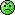Re: I.Q TEST: Let's See How Sound You Are In Quantitative Reasoning by matrix199(m): 9:35pm On Apr 12, 2020 Taymi:His solution is thrash Check the solution for the second puzzle on the first page. Take it easy young man or lady. Guess what, in the eyes of a monkey, humans are monkeys. Re: I.Q TEST: Let's See How Sound You Are In Quantitative Reasoning by Mechatronika(m): 9:39pm On Apr 12, 2020 Nobody should help Op in solving puzzle 2 o, I can't believe I've been waiting for olodo Op to come gimme the answer.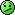Re: I.Q TEST: Let's See How Sound You Are In Quantitative Reasoning by matrix199(m): 9:42pm On Apr 12, 2020 It doesn't cost me anything to admit I'm wrong, if I was wrong. But you have to prove me wrong, to get me to admit I was wrong .It is called a puzzle, not an exam. So take a chill ok?! Re: I.Q TEST: Let's See How Sound You Are In Quantitative Reasoning by Taymi: 9:42pm On Apr 12, 2020 matrix199:Take it easy young man or lady. Guess what, in the eyes of a monkey, humans are monkeys.Sorry if it comes across as abuse oooooBecause you have seen Monkey, SMH for some assumed human.I only mean the solution isn’t taken by me Especially when I saw the second solution, I thrashed it immediately. My Opinion. Re: I.Q TEST: Let's See How Sound You Are In Quantitative Reasoning by matrix199(m): 9:43pm On Apr 12, 2020 Mechatronika:Op, your Puzzle 2 Analysis and answer is wrong, do it again the answer is 75!Can I lead you through it, or would you lead?

(1) (2) (3) (4) (5) (Reply)

(Go Up)

 Sections: politics (1) business autos (1) jobs (1) career education (1) romance computers phones travel sports fashion health religion celebs tv-movies music-radio literature webmasters programming techmarket Nairaland - Copyright © 2005 - 2021 Oluwaseun Osewa. All rights reserved. See How To Advertise. 119Disclaimer: Every Nairaland member is solely responsible for anything that he/she posts or uploads on Nairaland.